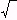Create a new printableGeometry
Math Worksheets

Sample - Click above to make a new math worksheet (PDF).
 Name _____________________________Date ___________________
The Pythagorean Theorem

1.
 a = 25 b = 59 c = ________

2.
 a = 48 b = 18 c = ________

3.
 a = ________ b = 56 c = 65

 4 * This is a pre-made sheet.Use the link at the top of the page for a printable page.
5.
a  =  21
b  =  ________
c  =
385

6.
a  =  ________
b  =  19
c  =442

7.
 a = 9 b = ________ c = 41

8.
a  =  16
b  =  ________
c  =
289

9.
 a = ________ b = 45 c = 53

10.
 a = 25 b = 17 c = ________

11.
a  =  ________
b  =  17
c  =818

12.
 a = 2 b = 20 c = ________

13.
 a = ________ b = 24 c = 25

14.
 a = 21 b = 25 c = ________

 15 * This is a pre-made sheet.Use the link at the top of the page for a printable page.
16.
 a = 25 b = 25 c = ________

17.
 a = ________ b = 15 c = 17

18.
a  =  4
b  =  ________
c  =
45